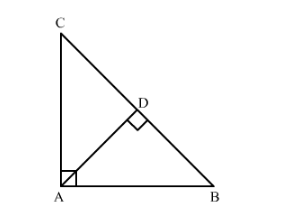# In an isosceles triangle ABC,

Question:

In an isosceles triangle ABC, if AB = AC = 25 cm and BC = 14 cm, the measure of altitude from A on BC is

(a) 20 cm
(b) 22 cm
(c) 18 cm
(d) 24 cm

Solution:

Given: In an isosceles triangle ABC, AB = AC = 25 cm and BC = 14cm.

To find: Measure of altitude from A on BCSince AD ⊥ BC, so BD = CD = BC2=14 cm2=7 cm

In right ∆ABD,

We got the result as $(d)$